# Dynamic programming

(diff) ← Older revision | Latest revision (diff) | Newer revision → (diff)

A branch of mathematics studying the theory and the methods of solution of multi-step problems of optimal control.

In dynamic programming of controlled processes the objective is to find among all possible controls a control that gives the extremal (maximal or minimal) value of the objective function — some numerical characteristic of the process. A multi-stage process is one the structure of which consists of several steps, or else a process in which the control is subdivided into a sequence of successive stages (steps), which usually correspond to different moments in time. In a number of problems the multi-stage nature is dictated by the process itself (for example, in the determination of the optimal dimensions of the steps of a multi-stage rocket or in finding the most economical way of flying an aircraft), but it may also be artificially introduced in order to be able to apply methods of dynamic programming. Thus, the meaning of "programming" in dynamic programming is making decisions and planning, while the word "dynamic" indicates the importance of time and of the sequence of execution of the operations in the process and methods under study.

The methods of dynamic programming are a part of the methods of operations research, and are utilized in optimal planning problems (e.g. in problems of the optimal distribution of available resources, in the theory of inventory management, replacement of equipment, etc.), and in solving numerous technical problems (e.g. control of successive chemical processes, optimal design of structural highway layers, etc.).

The basic idea will now be illustrated. Let the process of controlling a given systemconsist ofstages; at the-th step let the control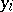convert the system from the state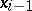attained during the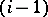-th step to the new state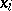. This transition process is realized by a given function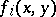, and the new state is determined by the values,: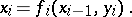Thus, the control operationsconvert the system from its initial stateinto the final state, whereand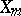are the sets of feasible initial and final states of. A possible way of stating the extremal problem is given below. The initial stateis known. The task is to select controls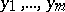so that the systemis converted to a feasible final state and, at the same time, the objective function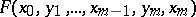attains its maximal value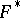, i.e.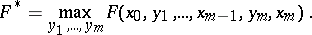An important feature of dynamic programming is that it is applicable to additive objective functions only. In the example above, this means that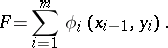Another requirement of the method is the absence of "after-effects" in the problem, i.e. the solutions (controls) selected for a given step may only affect the stateof the system at the moment. In other words, processes of the typeare not considered. Both these restrictions may be weakened, but only by unduly complicating the method.

Conventional methods of mathematical analysis are altogether inapplicable to problems of dynamic programming or else involve very laborious computations. The method of dynamic programming is based on the optimality principle formulated by R. Bellman: Assume that, in controlling a discrete system, a certain control on the discrete system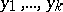, and hence the trajectory of states, have already been selected, and suppose it is required to terminate the process, i.e. to select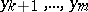(and hence); then, if the terminal part of the process is not optimal, i.e. if the maximum of the function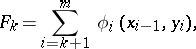is not attained by it, the process as a whole will not be optimal.

By using the optimality principle, the basic functional relationship is readily obtained. One determines the sequence of functions of the variable: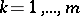. Here, the maximum is taken over all the control operations feasible at step. The relation defining the dependence ofon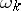is known as the Bellman equation. The meaning of the functions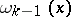is clear: If, in step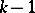, the system appears to be in state, thenis the maximum possible value of the function. Simultaneously with the construction of the functionsone also finds the conditional optimal controls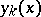at each step (i.e. the values of the optimal controls under the assumption that the system is in the stateat the step). The final optimal controls are found by successively computing the values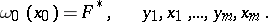The following property of the method of dynamic programming is evident from the above: It solves not merely one specific problem for a given, but all problems of this type, for all initial states. Since the numerical realization of the method of dynamic programming is laborious, i.e. requires a large storage capacity of the computer, it is expediently employed whenever certain typical problems have to be solved a large number of times (e.g. the optimum flight conditions for an aircraft under changing weather conditions). Even though dynamic programming problems are formulated for discrete processes, the method may often be successfully employed in solving problems with continuous parameters.

Dynamic programming furnished a novel approach to many problems of variational calculus.

An important branch of dynamic programming is constituted by stochastic problems, in which the state of the system and the objective function are affected by random factors. Such problems include, for example, optimal inventory control with allowance of random inventory replenishment. Controlled Markov processes are the most natural domains of application of dynamic programming in such cases.

The method of dynamic programming was first proposed by Bellman. Rigorous foundations of the method were laid by L.S. Pontryagin and his school, who studied the mathematical theory of control process (cf. Optimal control, mathematical theory of).

Even though the method of dynamic programming considerably simplifies the initial problems, its explicit utilization is usually very laborious. Attempts are made to overcome this difficulty by developing approximation methods.

How to Cite This Entry:
Dynamic programming. Encyclopedia of Mathematics. URL: http://encyclopediaofmath.org/index.php?title=Dynamic_programming&oldid=11658
This article was adapted from an original article by S.A. AshmanovV.G. Karmanov (originator), which appeared in Encyclopedia of Mathematics - ISBN 1402006098. See original article